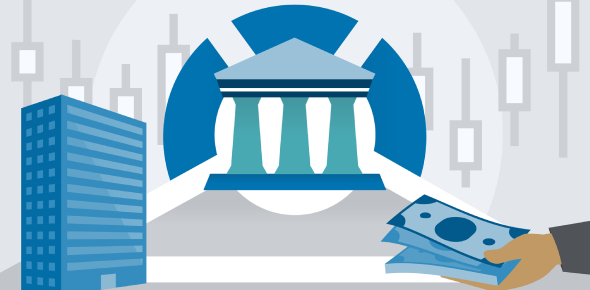MCQ Corporate Finance

20 Questions | Total Attempts: 4973Settings.

• 1.
At the interest rate of 15%, the 2 years discounting factor will be
• A.

0.7561

• B.

0.8697

• C.

0.9651

• D.

1.1225

• 2.
The firm's investment decision is also called as the
• A.

Financing Decision

• B.

Capital Budgeting Decision

• C.

Liquidity Decision

• D.

Dividend Decision

• 3.
As far as their value is concerned, the top priority group in company should be
• A.

Shareholders

• B.

Employees

• C.

Board of Directors

• D.

Governing Body

• 4.
Normally the Cost of Capital should be -----
• A.

Equal to IRR

• B.

Less than IRR

• C.

More than IRR

• D.

Corresponding to IRR

• 5.
Modigliani Miller Approach highlights the following aspect in dividend decisions
• A.

Rate of Dividend

• B.

Company tendency to retain earnings

• C.

Zero impact of market prices of shares

• D.

Shareholders' expectations

• 6.
From the following, which is not the direct middleman in the capital market ?
• A.

Rating Agencies

• B.

Broker

• C.

Banker

• D.

Trustee

• 7.
The term Over or Under Subscription is used in case of ---
• A.

Bank Financing

• B.

Public Issue

• C.

Non Performing Assets

• D.

Syndicate Financing

• 8.
If the present value of cash flow X is Rs.200 & that of Y is Rs.150, the combined present value of cash flows will be --
• A.

Rs.200

• B.

Rs.150

• C.

Rs.50

• D.

Rs.350

• 9.
Limited Liability is main feature of ---
• A.

Sole Proprietorship

• B.

Partnership

• C.

Corporations

• D.

N G O

• 10.
The Steel Authority Of India sold out 5% of their stake to private players & raised \$ 275 Million. This is popularly called as  --
• A.

• B.

• C.

Disinvestment

• D.

Foreign Direct Investment

• 11.
A formula of X-Y/Z is used for calculating the -- of Business Assets
• A.

Book Value

• B.

Market Value

• C.

Fair Value

• D.

Liquidation Value

• 12.
In ascertaining the EVA, the following component is to be considered.
• A.

Earnings Before Tax

• B.

Earnings After Tax & Interest

• C.

Earnings after Tax excluding Interest

• D.

Profits After Taxes

• 13.
Annuity is defined as -
• A.

Equal cash flows at equal intervals of time at a specific period

• B.

Equal cash flows at equal intervals of time forever

• C.

Unequal cash flows at equal intervals of time forever

• D.

Unequal cash flows at equal intervals of time for specific period

• 14.
The creditors of company are usually interested in observing the --
• A.

Liquidity Ratio

• B.

Solvency Ratio

• C.

Profitability Ratio

• D.

Turnover Ration

• 15.
From the following, which is not considered as the financial asset ?
• A.

Common Stock

• B.

Patents

• C.

Bonds

• D.

Preferred Stock

• 16.
Short Term Period in business is generally ..
• A.

Less than 3 Months

• B.

Less than 6 Months

• C.

Less than a year

• D.

Less than 5 years

• 17.
The Finance Controller is responsible for the following except
• A.

Preparation & interpretation of Financial Statements

• B.

Internal Auditing

• C.

Tax Management

• D.

• 18.
Financial leverage of the company is ascertaining by using..
• A.

Liquidity Ratio

• B.

Long Term Solvency Ratio

• C.

Turnover Ratio

• D.

Profitability Ratio

• 19.
Quick Ratio indicates the ..
• A.

Profitability of company

• B.

Cash position of company

• C.

Capital Structure of company

• D.

None of the above

• 20.
Any speculative stock having high probability in future is seem to be  ---- today.
• A.

High, Negative & Under-priced

• B.

High, Negative & Overpriced

• C.

High, Positive & Overpriced

• D.

Low, Negative & Overpriced

Related TopicsBack to top